Homework Help Question & Answers

# Include all relevant work please. 8. Find the general polynomial function of lowest possible degree with...

Include all relevant work please.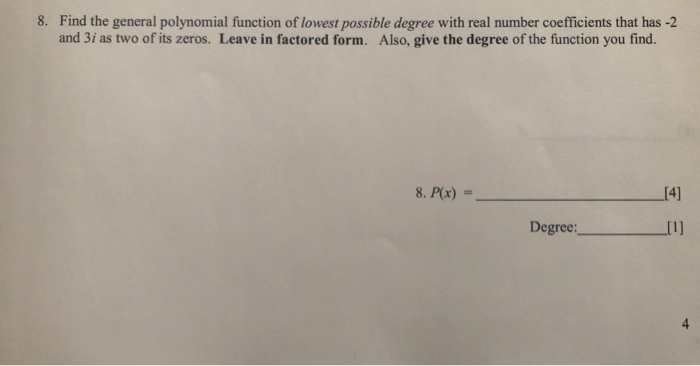8. Find the general polynomial function of lowest possible degree with real number coefficients that has -2 and 3i as two of its zeros. Leave in factored form. Also, give the degree of the function you find. 8. P(x)  Degree:

#### Homework Answers

Answer #1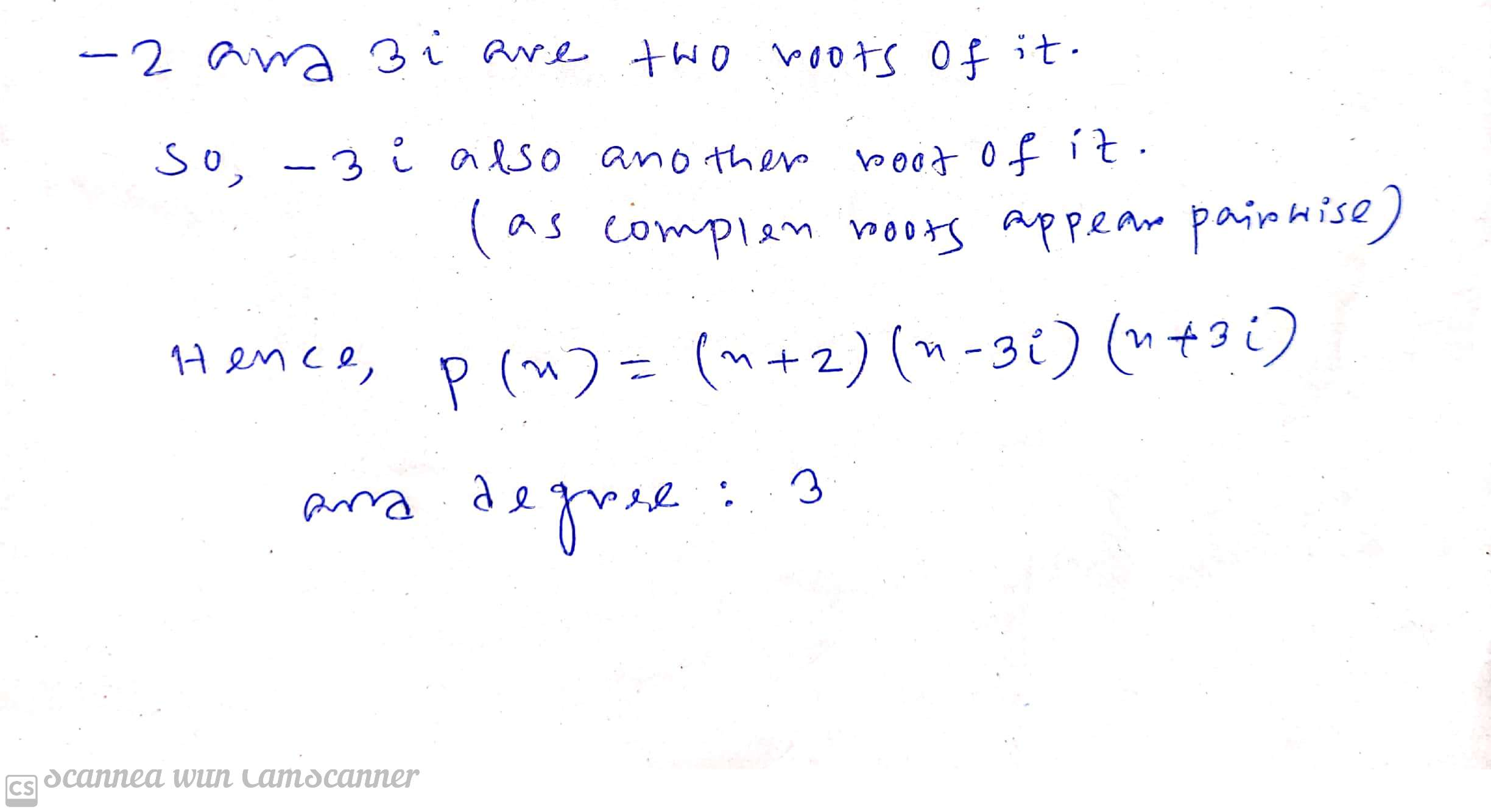as -2 ,3i , -3i are roots of it

So, factors are (x - (-2)) , ( x- (3i)) , (x - (-3i))

That is (x+2) , (x-3i) , (x+3i) are factor of P(x)

As their is 3 factor in it so degree must be 3

Know the answer?
Your Answer:

#### Post as a guest

Your Name:

What's your source?

#### Earn Coin

Coins can be redeemed for fabulous gifts.

Not the answer you're looking for? Ask your own homework help question. Our experts will answer your question WITHIN MINUTES for Free.
Similar Homework Help Questions
• ### Find a polynomial function P of the lowest possible degree, having real coefficients, a leading coefficient...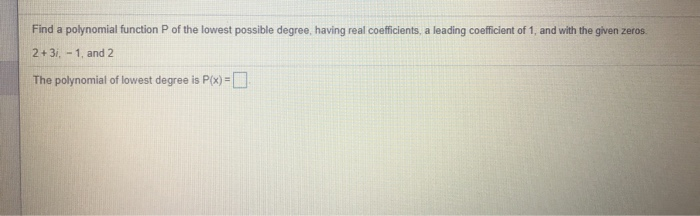Find a polynomial function P of the lowest possible degree, having real coefficients, a leading coefficient of 1, and with the given zeros. 2 + 3i. - 1 and 2 The polynomial of lowest degree is P(x) =

• ### Find a polynomial function of lowest degree with rational coefficients that has the given numbers as...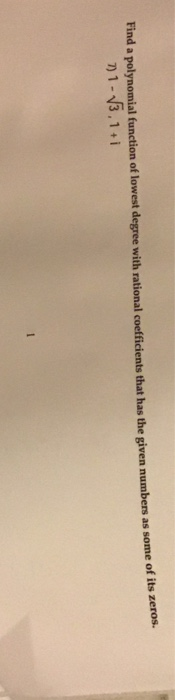Find a polynomial function of lowest degree with rational coefficients that has the given numbers as some of its zeros. 1-3,1+i

• ### 9-11 9. Find the polynomial of degree 4 whose graph is shown. (It is not necessary...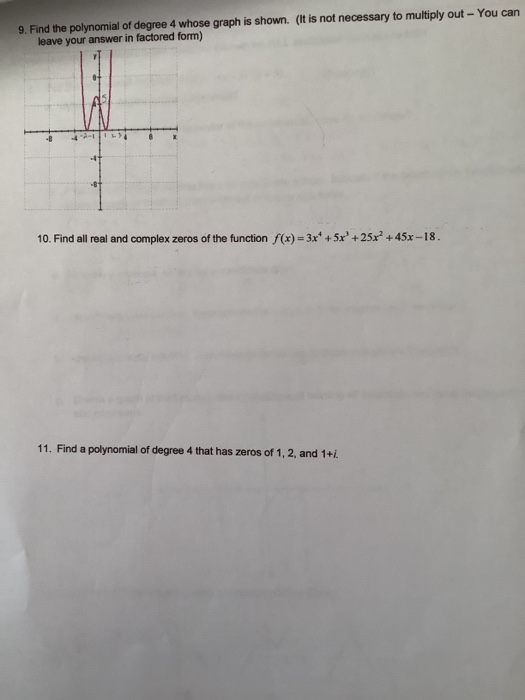9-11 9. Find the polynomial of degree 4 whose graph is shown. (It is not necessary to multiply out - You can leave your answer in factored form) 10. Find all real and complex zeros of the function f(x) = 3x* +5x' +25x +45x-18. 11. Find a polynomial of degree 4 that has zeros of 1, 2, and 1+i.

• ### write a polynomial function of least degree with integral coefficients whose zeros include 3 and 3i

write a polynomial function of least degree with integral coefficients whose zeros include 3 and 3i

• ### 4. Describe how to find the possible rational zeros of a polynomial function. Use the function...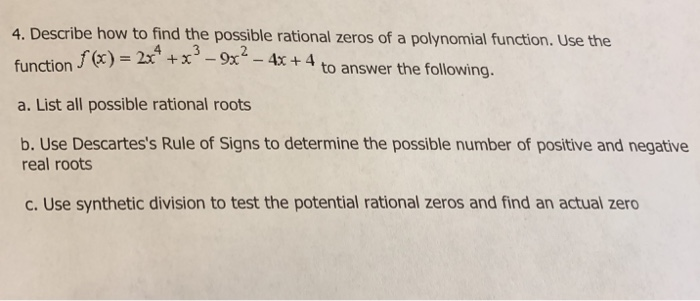4. Describe how to find the possible rational zeros of a polynomial function. Use the function f(x)-2x +13-9x2 a. List all possible rational roots b. Use Descartes's Rule of Signs to determine the possible number of positive and negative f(x) = 2t to answer the following. real roots c. Use synthetic division to test the potential rational zeros and find an actual zero d. Use the quotient from part(c) to find all the remaining zeros e. Rewrite f(x) in completely...

• ### QUESTION 6 0 poi Find a polynomial of lowest degree with only real coefficients and having...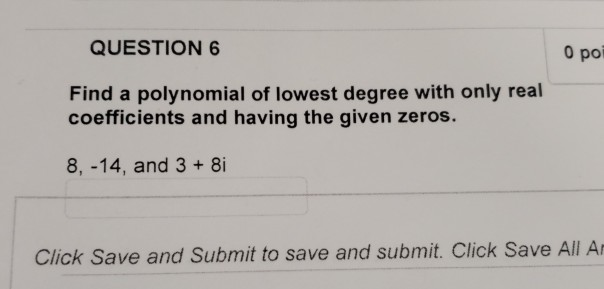QUESTION 6 0 poi Find a polynomial of lowest degree with only real coefficients and having the given zeros. 8,-14, and 3 8i Click Save and Submit to save and submit. Click Save All A

• ### please show work 13. 0/2 points Previous Answers StitzCA3 3.2.031. For the polynomial function given below,...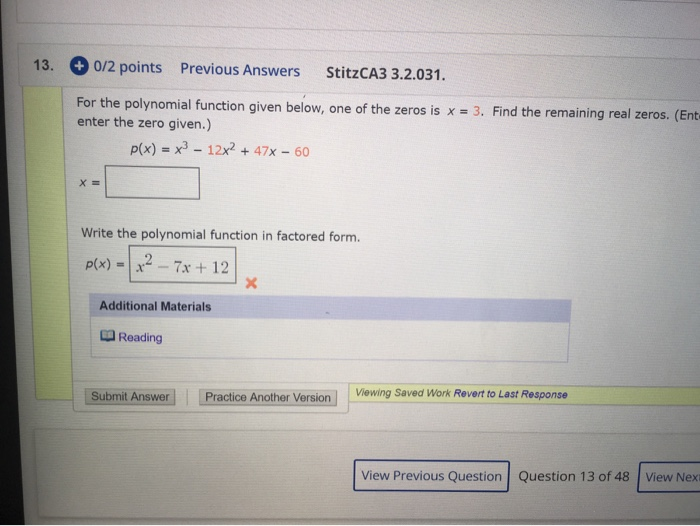please show work 13. 0/2 points Previous Answers StitzCA3 3.2.031. For the polynomial function given below, one of the zeros is x = 3. Find the remaining real zeros. (Ent enter the zero given.) P(x) = x3 - 12x2 + 47x - 60 x = Write the polynomial function in factored form. p(x) = x2 - 7x + 12 Additional Materials Reading Submit Answer Practice Another Version Viewing Saved Work Revert to Last Response View Previous Question Question 13 of...

• ### Find all zeros of the given polynomial function and write the polynomial in completely factored form....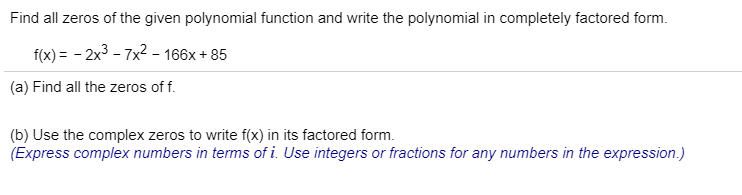Find all zeros of the given polynomial function and write the polynomial in completely factored form. f(x)- -2x3 -7x2 - 166x+85 (a) Find all the zeros of f (b) Use the complex zeros to write f(x) in its factored form. (Express complex numbers in terms of i. Use integers or fractions for any numbers in the expression.)

• ### Find a polynomial function of least degree having only real coefficients, a leading coefficient of 1,...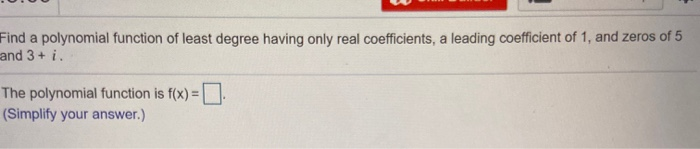Find a polynomial function of least degree having only real coefficients, a leading coefficient of 1, and zeros of 5 and 3 + i. The polynomial function is f(x) = (Simplify your answer.)

• ### Find a polynomial f(x) of degree 4 that has the following zeros. 2, 0, 7, -6...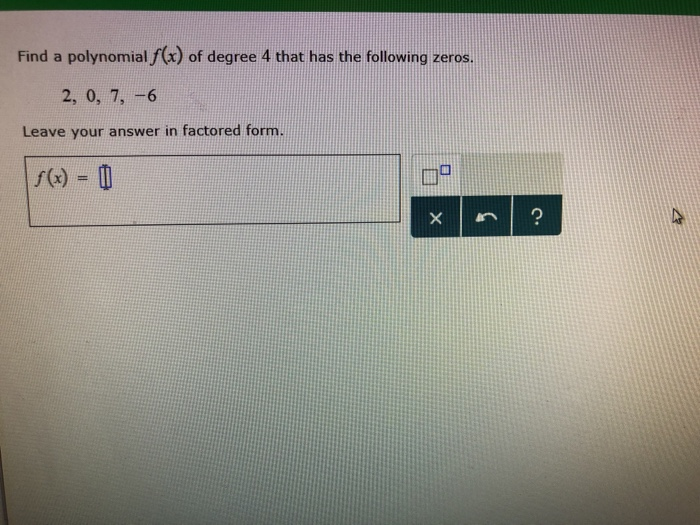Find a polynomial f(x) of degree 4 that has the following zeros. 2, 0, 7, -6 Leave your answer in factored form f(x)-Ф

Free Homework App

Scan Your Homework
to Get Instant Free Answers
Need Online Homework Help?

Get Answers For Free
Most questions answered within 3 hours.